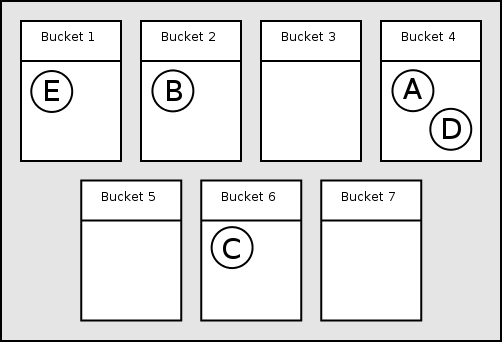#Boost C++ Libraries

...one of the most highly regarded and expertly designed C++ library projects in the world.

This is the documentation for an old version of boost. Click here for the latest Boost documentation.

## The Data Structure

The containers are made up of a number of 'buckets', each of which can contain any number of elements. For example, the following diagram shows an `unordered_set` with 7 buckets containing 5 elements, `A`, `B`, `C`, `D` and `E` (this is just for illustration, containers will typically have more buckets).In order to decide which bucket to place an element in, the container applies the hash function, `Hash`, to the element's key (for `unordered_set` and `unordered_multiset` the key is the whole element, but is referred to as the key so that the same terminology can be used for sets and maps). This returns a value of type `std::size_t`. `std::size_t` has a much greater range of values then the number of buckets, so that container applies another transformation to that value to choose a bucket to place the element in.

Retrieving the elements for a given key is simple. The same process is applied to the key to find the correct bucket. Then the key is compared with the elements in the bucket to find any elements that match (using the equality predicate `Pred`). If the hash function has worked well the elements will be evenly distributed amongst the buckets so only a small number of elements will need to be examined.

You can see in the diagram that `A` & `D` have been placed in the same bucket. When looking for elements in this bucket up to 2 comparisons are made, making the search slower. This is known as a collision. To keep things fast we try to keep collisions to a minimum.

Table 37.1. Methods for Accessing Buckets

Method

Description

`size_type bucket_count() const` The number of buckets.
```size_type max_bucket_count() const``` An upper bound on the number of buckets.
`size_type bucket_size(size_type n) const` The number of elements in bucket `n`.
`size_type bucket(key_type const& k) const` Returns the index of the bucket which would contain k
```local_iterator begin(size_type n);``` Return begin and end iterators for bucket `n`.
```local_iterator end(size_type n);```
```const_local_iterator begin(size_type n) const;```
`const_local_iterator end(size_type n) const;`
```const_local_iterator cbegin(size_type n) const;```
`const_local_iterator cend(size_type n) const;`

### Controlling the number of buckets

As more elements are added to an unordered associative container, the number of elements in the buckets will increase causing performance to degrade. To combat this the containers increase the bucket count as elements are inserted. You can also tell the container to change the bucket count (if required) by calling `rehash`.

The standard leaves a lot of freedom to the implementer to decide how the number of buckets are chosen, but it does make some requirements based on the container's 'load factor', the average number of elements per bucket. Containers also have a 'maximum load factor' which they should try to keep the load factor below.

You can't control the bucket count directly but there are two ways to influence it:

• Specify the minimum number of buckets when constructing a container or when calling `rehash`.
• Suggest a maximum load factor by calling `max_load_factor`.

`max_load_factor` doesn't let you set the maximum load factor yourself, it just lets you give a hint. And even then, the draft standard doesn't actually require the container to pay much attention to this value. The only time the load factor is required to be less than the maximum is following a call to `rehash`. But most implementations will try to keep the number of elements below the max load factor, and set the maximum load factor to be the same as or close to the hint - unless your hint is unreasonably small or large.

Table 37.2. Methods for Controlling Bucket Size

Method

Description

`X(size_type n)`

Construct an empty container with at least `n` buckets (`X` is the container type).

```X(InputIterator i, InputIterator j, size_type n)```

Construct an empty container with at least `n` buckets and insert elements from the range [`i`, `j`) (`X` is the container type).

`float load_factor() const`

The average number of elements per bucket.

`float max_load_factor() const`

Returns the current maximum load factor.

`float max_load_factor(float z)`

Changes the container's maximum load factor, using `z` as a hint.

```void rehash(size_type n)```

Changes the number of buckets so that there at least n buckets, and so that the load factor is less than the maximum load factor.

### Iterator Invalidation

It is not specified how member functions other than `rehash` affect the bucket count, although `insert` is only allowed to invalidate iterators when the insertion causes the load factor to be greater than or equal to the maximum load factor. For most implementations this means that insert will only change the number of buckets when this happens. While iterators can be invalidated by calls to `insert` and `rehash`, pointers and references to the container's elements are never invalidated.

In a similar manner to using `reserve` for `vector`s, it can be a good idea to call `rehash` before inserting a large number of elements. This will get the expensive rehashing out of the way and let you store iterators, safe in the knowledge that they won't be invalidated. If you are inserting `n` elements into container `x`, you could first call:

```x.rehash((x.size() + n) / x.max_load_factor() + 1);
```